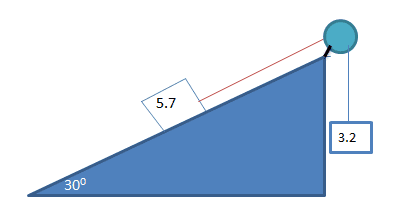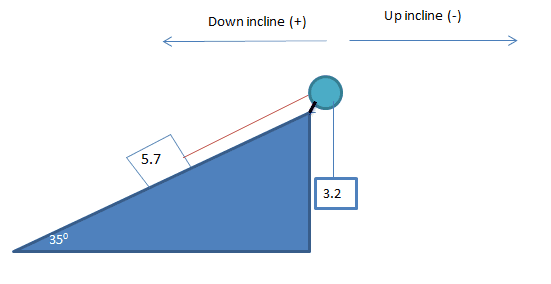# Forces in the triangle and pulley system

Two blocks are connected by a string-one on an incline, the rope draped over a pulley, and one hanging from the string. The smooth inclined surface makes an angle of 35 with the horizontal, and the block on the incline has a mass of 5.7 kg. The mass of the hanging block is 3.2kg. Find the direction and magnitude of the hanging block's acceleration.

Can someone explain to me why the smaller block should be represented as this equation?

For m: F(net) = ma = mg - T.

and not T - mg = ma? like the first one

Hi,

To fix the problem you are encountering, draw a diagram and set up the correct coordinate axis.**Woops that angle should be 35 degrees, but you get the picture :)

Can someone explain to me why the smaller block should be represented as this equation?

For m: F(net) = ma = mg - T.

and not T - mg = ma? like the first one

These equations are relative to the coordinate axis chosen. Essentially you have two choices in this problem: the system accelerating right, and the system accelerating left.

For example, let's choose 'acceleration of the system to the left' to be positive.

This means that all forces pointing left will be positive. We can now set up a system of equations for each box.

For the 5.7kg box on the incline, there are two forces acting on it that will cause the objects to go up or down the incline; since we chose 'accelerating left' as positive, all forces that will cause the box to slide down the incline, will be positive.

The two forces we have are: Fgx and FT
Fgx points down the incline so we make it positive (since we chose 'to the left' as positive').

FT points up the incline, and thus is assigned a negative value.

Therefore, our Fnet equation for the 5.7kg box will be: Fgx+(-FT)=ma

Apply this to the other box, then add the equations together and solve for a (the FT will cancel)

yes i had the FBD drawn and i understand i think how the larger mass should be moving the the left due to it's heavy weight but i am not getting why for the second mass tension is subtracted from the weight.

i drew a triangle perpendicular to the larger mass. indicating sin(35)mg is positive.

Last edited:
yes i had the FBD drawn and i understand i think how the larger mass should be moving the the left due to it's heavy weight but i am not getting why for the second mass tension is subtracted from the weight.

i drew a triangle perpendicular to the larger mass. indicating sin(35)mg is positive.

Careful, just because the mass is greater doesn't mean it is going to accelerate that way (the angle affects Fgx)

For example if the angle was 1o and there was no friction, the box would move right (think, there is no way it could move left)

I picked accelerating left as an arbitrary set up--you could have said 'to the right' is positive; however, the coordinates would be reversed.

i am not getting why for the second mass tension is subtracted from the weight.

Tension in the smaller mass is acting upwards, which would cause the system to move left, and therefore would be positive (from the axis we set up). Think, pulling on the rope holding the smaller mass will cause it to accelerate which way?**To the right will also be the same as saying the system accelerates towards the smaller mass

** To the left will also be the same as saying the system accelerates towards the larger mass.